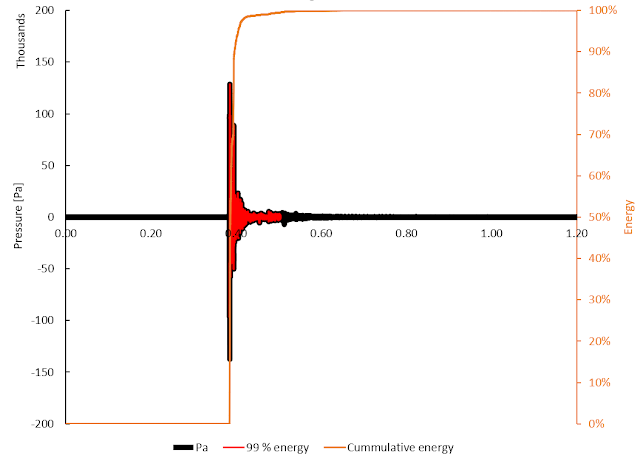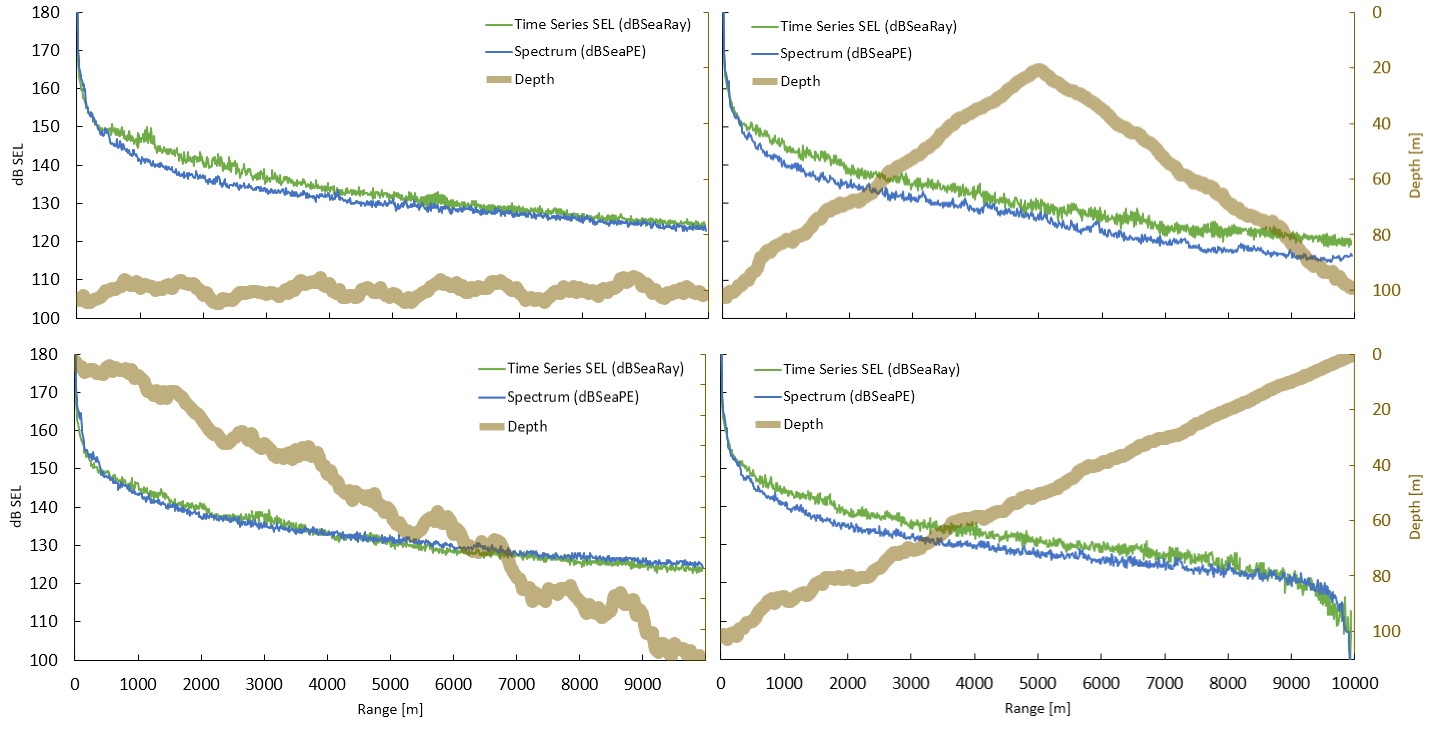## Bending the rules - how to make dBSea work smarter

### a description of a workaround for SEL calculations

As is often the case, my blog posts are based on conversations with dBSea users, and this is no different. In underwater noise propagation modelling we are often tasked with finding both zero-to-peak, peak-to-peak and impulse-SEL (z-p, p-p, iSEL) for scenarios involving impulsive noise sources such as impact pile driving or seismic surveys (see ISO 18405- 2017 for details on sound metrics ).

To calculate z-p and p-p levels we have to use a propagation method that allows us to take into account arrival times and phase for the arriving signal (Figure 1). In dBSea that means using the dBSeaRay solver that uses raytracing to find arrival times, transmission loss and phase of the impulse at all receiver positions in a scenario.Figure 1. Example of 4 ray paths from a source to a receiver, their associated travel time and delay. On the bottom the signal at thou source in red (short duration and high amplitude) and at the receiver (lower amplitude, but longer duration).

This type of calculation becomes very resource intensive as many paths need to be calculated for each receiving point and each receiving point has to store information about each part of the signal that has passed though it (timing, phase and transmission loss).

But while the z-p and p-p calculation have to be done using this rather slow approach, calculating the energy passing through a receiver location, or the Sound Exposure Level, can be done using one of the much faster solvers, here dBSeaPE (a Parabolic Equation type solver).

Two assumptions are used to justify the change in calculation method:
1. The average energy that passes through a receiver point is independent of the phase and arrival time of the individual ray path.
2. At frequencies over 1 kHz absorption is high (>1 dB/km) and we can disregard frequencies higher than this (dBSeaPE is much faster for lower frequencies)
We need 3 pieces of data from the original impulse:
1. The impulse SEL.
2. The length of the impulse (e.g. 99 % of energy used to determine this).
3. The spectrum of the impulse.
Those 3 can be taken from dBSea, or calculated in other programmes, e.g. MS Excel, Octave (highly recommended), MatLab etc.Figure 2. An impulse (black), associated cumulative energy (orange) and part of signal corresponding to 99 % of signal energy (red).

In dBSea we can now create a new "spectrum type" source with the spectrum from the impulse. The combined SEL of this new source needs to be corrected to the right SEL (adding band levels does not necessarily give the same level as from a time-series, due to lack of phase information). Lastly we set the "Assessment time" of the scenario to equal the length of the impulse (99 % energy).

Running the solve with dBSeaPE will now give us SEL results for the impulse, but calculation will be much quicker than with the raytracer.

Below are some example scenarios of the two methods side by side for comparison.
Green is dBSeaRay timeseries solve, blue is dBSeaPE spectrum solve.Figure 3. SEL as calculated by dBSeaRay and dBSeaPE for 4 different bathymetry profiles with a rough sandy sediment, 12.5-1000 Hz

Note that dBSeaPE can run these calculation in seconds while dBSeaRay will take ~10 minutes to run the calculations at equivalent resolution.

While results will not be identical a small statistical breakdown of variation in results show that they are not far apart (Figure 4 below).Figure 4. The difference between dBSeaPE and dBSeaRay when doing SEL calculation in 4 different scenarios. dBSeaPE tends to overpredict transmission losses compared to dBSeaRay by an average of 2.2 dB. Horizontal bars are ± 1 standard deviation.

Finally, I must clarify that this is a workaround, which achieves faster calculation times, but might increase the risk of wrong results if you do not clearly understand the underlying theory.

So - please use the above information as you please, but keep in mind that making mistakes will get you in trouble!

References: